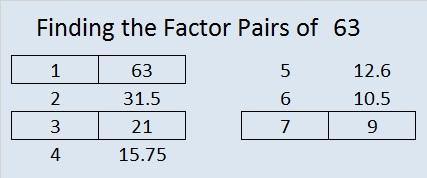# 63 and Twelve Quick Clues

63 is a composite number. 63 = 1 x 63, 3 x 21, or 7 x 9. Factors of 63: 1, 3, 7, 9, 21, 63. Prime factorization: 63 = 3 x 3 x 7, which can also be written 63 = 3² x 7.Sometimes 63 is a clue in the FIND THE FACTORS puzzles. Even though it has other factors, the only multiplication fact we use is 7 x 9 = 63.All Previously Published Puzzles